# High School Math : How to find the length of the side of a right triangle

## Example Questions

1 3 Next →

### Example Question #141 : Act Math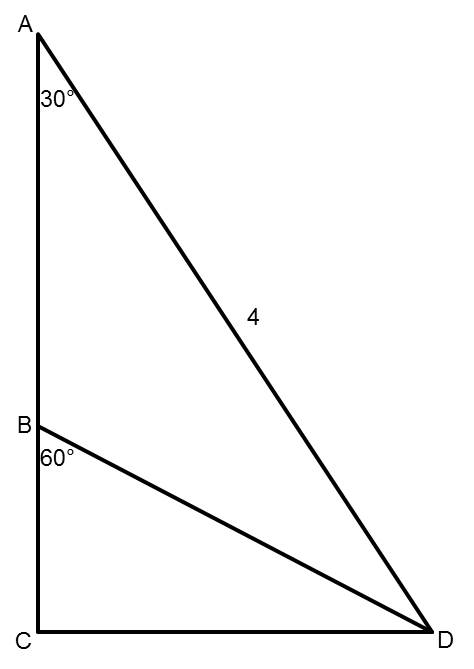Points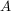,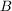, and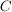are collinear (they lie along the same line).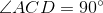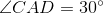,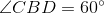,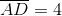Find the length of segment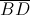.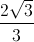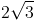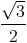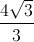The length of segmentisNote that triangles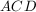and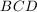are both special, 30-60-90 right triangles. Looking specifically at triangle, because we know that segment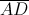has a length of 4, we can determine that the length of segmentis 2 using what we know about special right triangles. Then, looking at trianglenow, we can use the same rules to determine that segmenthas a length of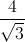which simplifies to.